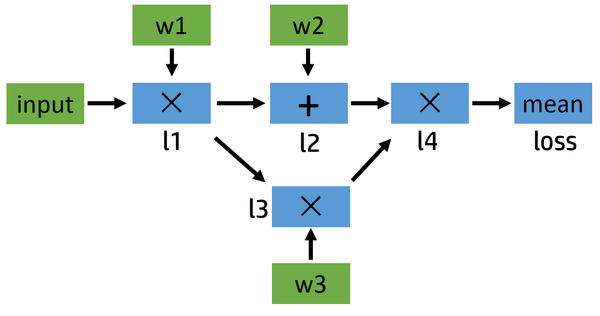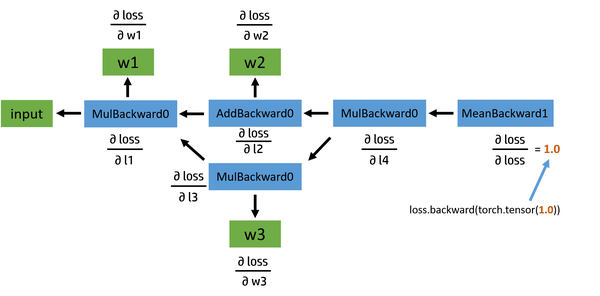PyTorch 作为一个深度学习平台，在深度学习任务中比 NumPy 这个科学计算库强在哪里呢？我觉得一是 PyTorch 提供了自动求导机制，二是对 GPU 的支持。由此可见，自动求导 (autograd) 是 PyTorch，乃至其他大部分深度学习框架中的重要组成部分。

1. 计算图
2. 一个具体的例子
3. 叶子张量
4. inplace 操作
5. 动态图，静态图

## 计算图

a = torch.tensor(2.0, requires_grad=True)
b = a.exp()
print(b)
# tensor(7.3891, grad_fn=<ExpBackward>)

## 一个具体的例子

l1 = input x w1
l2 = l1 + w2
l3 = l1 x w3
l4 = l2 x l3
loss = mean(l4)input = torch.ones([2, 2], requires_grad=False)

l1 = input * w1
l2 = l1 + w2
l3 = l1 * w3
l4 = l2 * l3
loss = l4.mean()

# tensor(2.) None None

# tensor([[2., 2.],
#         [2., 2.]]) None <MulBackward0 object at 0x000001EBE79E6AC8>

# tensor(40.) None <MeanBackward0 object at 0x000001EBE79D8208>input = [1.0, 1.0, 1.0, 1.0]
w1 = [2.0, 2.0, 2.0, 2.0]
w2 = [3.0, 3.0, 3.0, 3.0]
w3 = [4.0, 4.0, 4.0, 4.0]

l1 = input x w1 = [2.0, 2.0, 2.0, 2.0]
l2 = l1 + w2 = [5.0, 5.0, 5.0, 5.0]
l3 = l1 x w3 = [8.0, 8.0, 8.0, 8.0]
l4 = l2 x l3 = [40.0, 40.0, 40.0, 40.0]
loss = mean(l4) = 40.0

$\frac{\partial \, loss}{\partial \, w_2} = \frac{\partial \, loss}{\partial \, l_4} \frac{\partial \, l_4}{\partial \, l_2} \frac{\partial \, l_2}{\partial \, w_2} = [2.0, 2.0, 2.0, 2.0]$ ，其和为 8。

loss.backward()

# tensor(28.) tensor(8.) tensor(10.)
# None None None None None

## 叶子张量

a = torch.ones([2, 2], requires_grad=True)
print(a.is_leaf)
# True

b = a + 2
print(b.is_leaf)
# False
# 因为 b 不是用户创建的，是通过计算生成的

# 和前边一样
# ...
loss = l4.mean()

loss.backward()

# tensor(1.)
# tensor([[0.2500, 0.2500],
#         [0.2500, 0.2500]])
# tensor([[7., 7.],
#         [7., 7.]])

# 和前边一样
# ...
loss = l4.mean()

loss.backward()

#         [0.2500, 0.2500]])
#         [7., 7.]])

# None
# loss 的 grad 在 print 完之后就被清除掉了

## inplace 操作

RuntimeError: one of the variables needed for gradient computation has been modified by an inplace operation: balabala...

# 情景 1
a = a.exp()

# 情景 2
a = 10

# 我们要用到 id() 这个函数，其返回值是对象的内存地址
# 情景 1
a = torch.tensor([3.0, 1.0])
print(id(a)) # 2112716404344
a = a.exp()
print(id(a)) # 2112715008904
# 在这个过程中 a.exp() 生成了一个新的对象，然后再让 a
# 指向它的地址，所以这不是个 inplace 操作

# 情景 2
a = torch.tensor([3.0, 1.0])
print(id(a)) # 2112716403840
a = 10
print(id(a), a) # 2112716403840 tensor([10.,  1.])
# inplace 操作，内存地址没变

PyTorch 是怎么检测 tensor 发生了 inplace 操作呢？答案是通过 tensor._version 来检测的。我们还是来看个例子：

a = torch.tensor([1.0, 3.0], requires_grad=True)
b = a + 2
print(b._version) # 0

loss = (b * b).mean()
b = 1000.0
print(b._version) # 1

loss.backward()
# RuntimeError: one of the variables needed for gradient computation has been modified by an inplace operation ...

RuntimeError: leaf variable has been moved into the graph interior

a = torch.tensor([10., 5., 2., 3.], requires_grad=True)
print(a, a.is_leaf)
# tensor([10.,  5.,  2.,  3.], requires_grad=True) True

a[:] = 0
print(a, a.is_leaf)
# tensor([0., 0., 0., 0.], grad_fn=<CopySlices>) False

loss = (a*a).mean()
loss.backward()
# RuntimeError: leaf variable has been moved into the graph interior

a = torch.tensor([10., 5., 2., 3.], requires_grad=True)
a.add_(10.) # 或者 a += 10.
# RuntimeError: a leaf Variable that requires grad has been used in an in-place operation.

# 方法一
a = torch.tensor([10., 5., 2., 3.], requires_grad=True)
print(a, a.is_leaf, id(a))
# tensor([10.,  5.,  2.,  3.], requires_grad=True) True 2501274822696

a.data.fill_(10.)
# 或者 a.detach().fill_(10.)
print(a, a.is_leaf, id(a))
# tensor([10., 10., 10., 10.], requires_grad=True) True 2501274822696

loss = (a*a).mean()
loss.backward()
# tensor([5., 5., 5., 5.])

# 方法二
a = torch.tensor([10., 5., 2., 3.], requires_grad=True)
print(a, a.is_leaf)
# tensor([10.,  5.,  2.,  3.], requires_grad=True) True

a[:] = 10.
print(a, a.is_leaf)
# tensor([10., 10., 10., 10.], requires_grad=True) True

loss = (a*a).mean()
loss.backward()
# tensor([5., 5., 5., 5.])

## 动态图，静态图

# 这是一个关于 PyTorch 是动态图的例子：
b = a * a
loss = b.mean()

loss.backward() # 正常
loss.backward() # RuntimeError

# 第二次：从头再来一遍
b = a * a
loss = b.mean()
loss.backward() # 正常